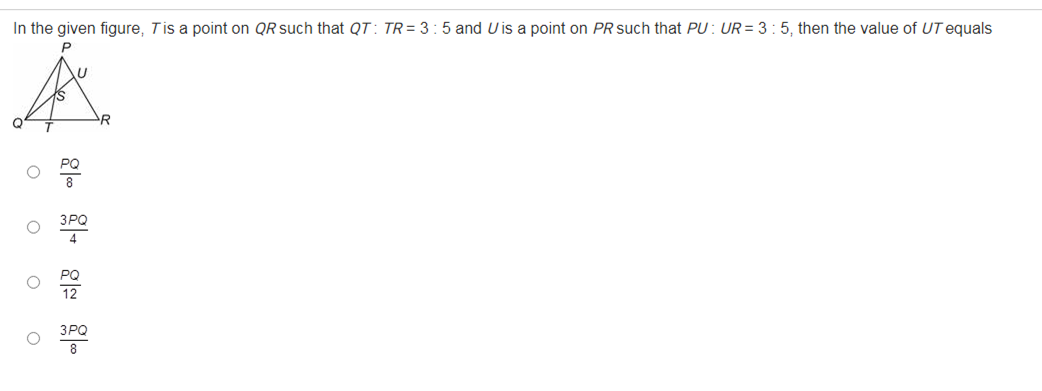Request a call backAsked by seeni2005 | 20 Aug, 2020, 02:05: PMExpert Answer

Given: QT : TR = 3 : 5

QT/TR = 3/5

QT/TR +  1 = 3/5 + 1

(QT + TR)/TR = (3 + 5)/5

QR/TR = 8/5

PU : UR = 3 : 5  ... (Given)

PU/UR = 3/5

PU/UR + 1 = 3/5 + 1

PR/UR  = 8/5

Comparing  Δ UTR  &  ΔPQR

QR/TR = PR/UR  = 8/5

∠R is common

Therefore, Δ UTR similar to ΔPQR .... (SAS similarity criteria )

UT/PQ  =  UR/PR

UT/PQ  =  5/8

UT = (5/8) PQ

Answered by Renu Varma | 24 Aug, 2020, 12:03: PM